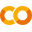Swiftpack.co - Package - cgarciae/NDArray

# NDArray

NDArray is a multidimensional array library written in Swift that aims to become the equivalent of `numpy` in Swift's emerging data science ecosystem. This project is in a very early stage and has a long but exciting road ahead!

## Goals

1. Have an efficient multidimensional array interface with common things like indexing, slicing, broadcasting, etc.
2. Make `NDArray` and its operations `differentiable` so its usable along with Swift for TensorFlow.
3. Create specialized implementations of linear algebra operations for NDArrays containing numeric types using BLAS, LAPACK, Accelerate, or MLIR depending on the environment.

## TutorialsTutorial | Last Updated | -------- | ------------ | Basic API | August 13 2019 |

## Installation

You can install it using SwiftPM:

``````.package(url: "https://github.com/cgarciae/NDArray", from: "0.0.19")
``````

It might work on other compatible package managers. This package is only tested in Swift 5.1, compatibility with previous version is not guaranteed.

## Example

`NDArray` is a generic container type just like `Array` with the difference that its multidimensional. If its elements conform to certain protocols then certain methods and operators like `+`, `-`, `*`, etc, can be used to efficiently perform computations of the whole collection.

``````import NDArray

let a = NDArray<Int>([
[1, 2, 3],
[4, 5, 6],
])
let b = NDArray<Int>([
[7, 8, 9],
[10, 11, 12],
])

print((a + b) * a)
/*
NDArray<Int>[2, 3]([
[8, 20, 36],
[56, 80, 108],
])
*/
``````

Here we see that the outcome of `(a + b) * a` is also and `NDArray` of `Int` with shape `[2, 3]`. To use operators like `+` and `*` with NDArrays containing your custom types you just have to make them conform to the proper protocols. For example:

``````import NDArray

struct Point: AdditiveArithmetic {
let x: Float
let y: Float
...
}

let a = NDArray<Point>([Point(x: 1, y: 2), Point(x: 2, y: 3)])
let b = NDArray<Point>([Point(x: 4, y: 5), Point(x: 6, y: 7)])

print(a + b)
/*
NDArray<Point>([Point(x: 5.0, y: 7.0), Point(x: 8.0, y: 10.0)])
*/
``````

You can also apply generic transformations over the data, the previous could have been written as:

``````elementwise(a, b, apply: +)
// or
elementwise(a, b) { \$0 + \$1 }
``````

For heavy computation you can use the parallelized version:

``````elementwiseInParallel(a, b) {
// code
return c
}
``````

In the future `NDArray` should be able to estimate the best strategy (serial/parallelized) based on the type and size of the data.

## Goals Discussion

Except for the Basic API, NDArray's Automatic Differentiation and Linear Algebra Optimization capabilities should be opt-in so all users can have access to the library regardless of their environment, i.e. iOS developers should be able to use it even if they don't have access to TensorFlow's compiler or the Lineal Algebra infrastructure.

#### Basic API

The first goal is the definition of the library's basic API using pure Swift with no extra optimization or differentiable capabilities. iOS/OSX developers should be able to use the basic API without additional setup. It will also be important to keeping the NDArray's API in close coordination with Swift for TensorFlow's Tensor API to promote knowledge reuse and free documentation if possible.

#### Automatic Differentiation

The second goal is an obvious must have, Swift for TensorFlow's compiler with automatic differentiation is arguably the future of ML and we should use it.

#### Linear Algebra Optimization

The third goal is what you would expect from any HPC numeric library, the strategy would be to specialize functions/operations for numeric types by using BLAS, LAPACK, Accelerate, or MLIR to speed computation. On the other hand, if successfully integrated with MLIR, BLAS and LAPACK might not be necessary and NDArray could easily become one of the most performant numeric libraries out there.

## Roadmap

##### 0.1: Basic API
• [x] Indexing
• [x] Dimension Slicing
• [x] Dimension Filtering by Indexes
• [x] Dimension Masking
• [x] SqueezeAxis
• [x] NewAxis
• [x] Assignment
• [x] Broadcasting
• [x] Pretty Print
• [x] Elementwise Operations
• [x] Basic Operators: `+`, `-`, `*`, `\`
• [ ] Reduction Operations
• [ ] Subscript Bound Checks
• [ ] Fancy Indexing
• [ ] > 95% Coverage
• [ ] Documentation
##### 0.2: Differentiable Programming

This can actually be started at any point, although it wont be that useful until various operations like `dot` or reductions like `sum` or `mean` are implemented.

• [ ] Conform `NDArray` to `Differentiable`
• [ ] Make `NDArrays` operations differentiable.
##### 0.3: Linear Algebra Optimization
• [x] Link BLAS and LAPACK
• [ ] Specialize operations using BLAS, LAPACK, Accelerate, or MLIR
• [ ] `dot`
• [ ] ...

## Meta

Cristian Garcia – cgarcia.e88@gmail.com

Distributed under the MIT license. See LICENSE for more information.

## Github

 link Stars: 33
##### Help us keep the lights onTotal: 0

NDArrayProtocol

## 0.0.18 - Aug 16, 2019

Remove scalar overloads from elementwiseInParallel

## 0.0.17 - Aug 16, 2019

remove scalar overloading function of elementwise

## 0.0.16 - Aug 13, 2019

`..` operator